##### The Japanese Bonsai specialist
Direct order Contact Help / Services Newsletter# Habenaria radiata white egret orchid 1 bulb

Sagisoo orchid orchidee colombe pecteilis radiata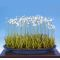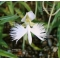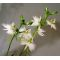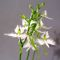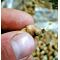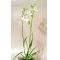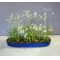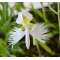ref. : 4718

14,00

This item is temporarily unavailableNotify availability

###### Description

1 bulb +- 3/8 mm. Sold and delivered with akadama soil for potting the bulbs and an plastic  bonsai pot, Ø + - 10 cm. Flower height 25 / 30 cm. It's a vivacious plant with fleshy roots to cultivate in a pot.

A Japanese species of orchid that flowers in a slightly fragrant cluster that makes an extremely romantic gift. These delicate mini Habenaria radiata fringed orchids have a beautiful story attached to them. The legend says that, during the civil wars in Japan, a girl in Setagaya castle, Tokyo, sent a letter to her lover in the war. She gave it to a white egret to carry it to him but the bird was shot down and where it fell on the ground this orchid flower grew. Today this flying egret orchid remains a symbol of Setagaya castle.

Cultivation: The foliage disappears in winter. During the period of rest throughout the colder months it's easy to cultivate this plant in a cold greenhouse without too much water. In April leaves will start to appear and at the start of summer you'll be rewarded with clusters of flowers that last between two and four weeks. Fertilise after flowering until the end of autumn.

These orchids are imported from Japan with a C.I.T.E.S licence.

#orchid 5.6 #egret 4.5 #that 4.4 #with 4.4 #habenaria 3.9 #radiata 3.7 #white 3.5 #bulb 3.4 #this 3.4 #cultivate 2.9

Formule
(( ROUND((CHAR_LENGTH(b.article_nom)-CHAR_LENGTH(REPLACE(b.article_nom, 'orchid', '')))/LENGTH('orchid')) + ROUND((CHAR_LENGTH(b.article_description)-CHAR_LENGTH(REPLACE(b.article_description, 'orchid', '')))/LENGTH('orchid')) ) * 5.6) + (( ROUND((CHAR_LENGTH(b.article_nom)-CHAR_LENGTH(REPLACE(b.article_nom, 'egret', '')))/LENGTH('egret')) + ROUND((CHAR_LENGTH(b.article_description)-CHAR_LENGTH(REPLACE(b.article_description, 'egret', '')))/LENGTH('egret')) ) * 4.5) + (( ROUND((CHAR_LENGTH(b.article_nom)-CHAR_LENGTH(REPLACE(b.article_nom, 'that', '')))/LENGTH('that')) + ROUND((CHAR_LENGTH(b.article_description)-CHAR_LENGTH(REPLACE(b.article_description, 'that', '')))/LENGTH('that')) ) * 4.4) + (( ROUND((CHAR_LENGTH(b.article_nom)-CHAR_LENGTH(REPLACE(b.article_nom, 'with', '')))/LENGTH('with')) + ROUND((CHAR_LENGTH(b.article_description)-CHAR_LENGTH(REPLACE(b.article_description, 'with', '')))/LENGTH('with')) ) * 4.4) + (( ROUND((CHAR_LENGTH(b.article_nom)-CHAR_LENGTH(REPLACE(b.article_nom, 'habenaria', '')))/LENGTH('habenaria')) + ROUND((CHAR_LENGTH(b.article_description)-CHAR_LENGTH(REPLACE(b.article_description, 'habenaria', '')))/LENGTH('habenaria')) ) * 3.9) + (( ROUND((CHAR_LENGTH(b.article_nom)-CHAR_LENGTH(REPLACE(b.article_nom, 'radiata', '')))/LENGTH('radiata')) + ROUND((CHAR_LENGTH(b.article_description)-CHAR_LENGTH(REPLACE(b.article_description, 'radiata', '')))/LENGTH('radiata')) ) * 3.7) + (( ROUND((CHAR_LENGTH(b.article_nom)-CHAR_LENGTH(REPLACE(b.article_nom, 'white', '')))/LENGTH('white')) + ROUND((CHAR_LENGTH(b.article_description)-CHAR_LENGTH(REPLACE(b.article_description, 'white', '')))/LENGTH('white')) ) * 3.5) + (( ROUND((CHAR_LENGTH(b.article_nom)-CHAR_LENGTH(REPLACE(b.article_nom, 'this', '')))/LENGTH('this')) + ROUND((CHAR_LENGTH(b.article_description)-CHAR_LENGTH(REPLACE(b.article_description, 'this', '')))/LENGTH('this')) ) * 3.4) + (( ROUND((CHAR_LENGTH(b.article_nom)-CHAR_LENGTH(REPLACE(b.article_nom, 'bulb', '')))/LENGTH('bulb')) + ROUND((CHAR_LENGTH(b.article_description)-CHAR_LENGTH(REPLACE(b.article_description, 'bulb', '')))/LENGTH('bulb')) ) * 3.4) + (( ROUND((CHAR_LENGTH(b.article_nom)-CHAR_LENGTH(REPLACE(b.article_nom, 'cultivate', '')))/LENGTH('cultivate')) + ROUND((CHAR_LENGTH(b.article_description)-CHAR_LENGTH(REPLACE(b.article_description, 'cultivate', '')))/LENGTH('cultivate')) ) * 2.9)

## Secure payment## Delivery

Our logistic partners :04 74 55 23 48
Pépinière MAILLOT-BONSAÏ
Le Bois Frazy
01990 RELEVANT - FRANCE
on appointment